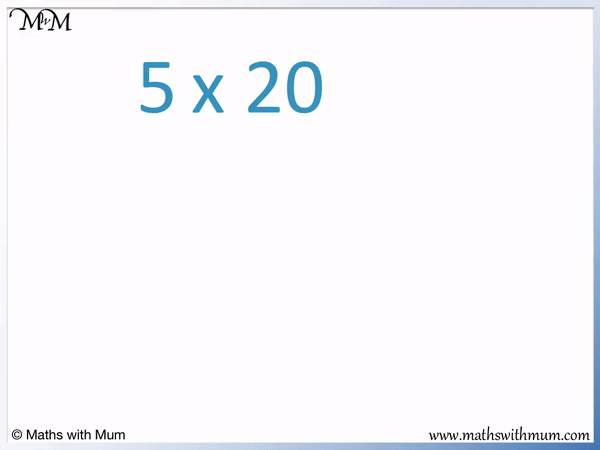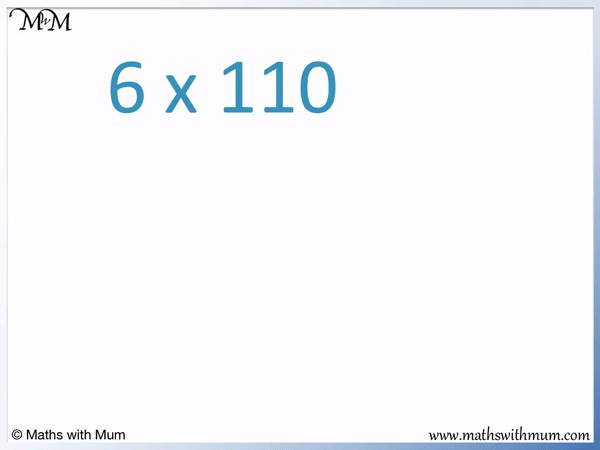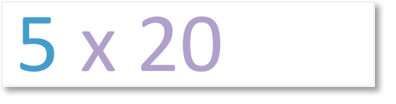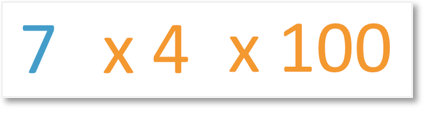# Multiplying by Multiples of 10 and 100

Multiplying by Multiples of 10 and 100#### Multiplying by a Multiple of 10• A multiple of 10 is a number in the 10 times table.
• To multiply a whole number by 10, simply write a zero digit on the end of it.
• To multiply a number by a multiple of 10, multiply the number digits in front of the 0 digit and then write a 0 on the end.
• 20 is a multiple of 10. 20 is 2 × 10.
• To work out 5 × 20, we can work out 5 × 2 × 10.
• 5 × 2 = 10 and now we just need to multiply by 10.
• To multiply 10 by 10, we simply write a 0 digit on the end.
• 10 × 10 = 100 and so, 5 × 20 = 100.

#### Multiplying by a Multiple of 100• A multiple of 100 is a number in the 100 times table.
• To multiply a whole number by 100, simply write two 0 digits on the end of it.
• To multiply a number by a multiple of 100, multiply the number by the digits in front of the two 0 digits and then write two 0 digits on the end.
• 400 is a multiple of 100. 400 = 4 × 100.
• To work out 7 × 400, we can work out 7 × 4 × 100.
• 7 × 4 = 28 and now we just need to multiply by 100.
• To multiply 28 by 100 we simply write two 0 digits on the end.
• 28 × 100 = 2800 and so, 7 × 400 = 2800.

Multiply the number by the first digits of the multiple of 10 or 100.

Write the same number of zeros on the end of the answer as there are in 10 or 100.• 110 is a multiple of 10 because it ends in one 0 digit.
• 110 = 11 × 10 so to multiply 6 by 110, we will multiply 6 × 11 × 10.
• 6 × 11 = 66 and we will multiply by 10 by writing a 0 on the end.
• 66 × 10 = 660 and so, 6 × 110 = 660.Supporting Lessons# Multiplying by Multiples of 10 and 100

## What is a Multiple of 10?

A multiple of 10 is a number in the 10 times table. Multiples of 10 will always have a last digit of 0. The first few multiples of 10 are 10, 20, 30, 40, 50, 60, 70, 80, 90 and 100.

We can recognise multiples of 10 because they are numbers that end in at least one 0 digit.

## What is a Multiple of 100?

A multiple of 100 is a number in the 100 times table. Multiples of 100 will always have two 0 digits as their last two digits. The first few multiples of 100 are 100, 200, 300, 400, 500, 600, 700, 800, 900 and 1000.

We can recognise multiples of 100 because they are numbers that end in at least two 0 digits.

## How to Multiply by Multiples of 10

To multiply a whole number by a multiple of 10, follow these steps:

1. Multiply the whole number by the digits in front of the last 0 in the multiple of 10.
2. Multiply this result by 10 by writing a 0 digit on the end.

For example, we will multiply 5 × 20.20 is a multiple of 10 because it ends in a 0 digit.

We can write multiples of 10 as the digits in front of the zero digit multiplied by 10. For example, 20 = 2 × 10.Multiplying by 20 is the same as multiplying by 2 and then by 10.

To multiply 5 × 20, we multiply 5 × 2 × 10.5 × 2 = 10.10 × 10 = 100. To multiply a whole number by 10, simply write a 0 digit on the end. 10 becomes 100.5 × 20 = 5 × 2 × 10 = 10 × 10 = 100. Therefore 5 × 20 = 100.

To multiply by a multiple of 10, simply multiply the digits ignoring the last 0 digit and then write the 0 digit back in at the end.

Because 5 × 2 = 10, 5 × 20 = 100.Here is another example of multiplying by a multiple of 10. We have 6 x 110.

110 is a multiple of 10 because it ends in a 0.6 x 11 = 66 and so, 6 x 110 = 660.

## How to Multiply by Multiples of 100

To multiply a whole number by a multiple of 100, follow these steps:

1. Multiply the whole number by the digits in front of the last two 0 digits in the multiple of 100.
2. Multiply this result by 100 by writing two 0 digits on the end.

For example, here is 7 × 400. 400 is a multiple of 100 because it ends in 2 zero digits.We multiply 7 by 4 and then write two 0 digits on the end to multiply by 100.

Multiplying by 400 is the same as multiplying by 4 and then 100.7 x 400 is the same as 7 x 4 x 100.4 x7 = 28. We now need to multiply by 100.

To multiply a whole number by 100, simply write two 0 digits on the end.28 x 100 = 2800. Therefore 7 x 400 = 2800.Because 7 x 4 = 28, 7 x 400 = 2800.

Here is another example of multiplying by a multiple of 100. We have 12 x 500.

500 is a multiple of 100 because it ends in 2 zero digits.12 x 5 = 60 and so, 12 x 500 = 6000.

We simply write 2 more zeros on the end of 60 to make 6000.Now try our lesson on Multiplying by 4 and 8 Using Doubling where we learn how to use doubling to multiply by 4 and 8.error: Content is protected !!